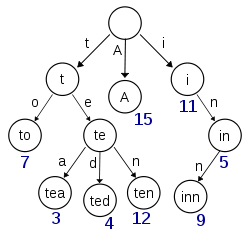# 结构的艺术：模糊查询

①：单词的长度；
②：每个位置的字母。typedef struct TrieTree
{
int isStr;    //标记这个单词是否存在
struct TrieTree * next[N];//N为26
}Trie;

int Insert(char * str, Trie * root)
{
int len = strlen(str);
Trie * temp = root;
for (int i = 0; i < len; i++)
{
int index = str[i]-'a';
if (temp->next[index] == NULL)
{
temp->next[index] = (Trie *)malloc(sizeof(Trie));
temp = temp->next[index];
temp->isStr = 0;
for (int i = 0; i < N; i++)
{
temp->next[i] = NULL;
}
}
else
{
temp = temp->next[index];
}
}
if (temp->isStr == 1)
return 0;
temp->isStr = 1;
return 1;
}

①：找到字符串对应的节点，直接将该节点对应的标记值改为0，表示该字符串不在树中。
②：找到字符串对应的节点，检查该节点是否有子孙节点，如果有则将该节点对应的标记值改为0，如果没有，就得删除该节点，并且如果该节点的祖先节点对其余节点不产生影响的话，要递归删除该节点的祖先节点。

int Delete(char * str, Trie * root)
{
int len = strlen(str);
Trie * temp = root;
for (int i = 0; i < len; i++)
{
int index = str[i]-'a';
if (temp->next[index])
temp = temp->next[index];
else
return 0;
}
if (temp->isStr == 0)   return 0;
temp->isStr = 0;
return 1;
}

int Fuzzys(char * str, Trie * root)
{
int len = strlen(str);
Trie * temp = root;
for (int i = 0; i < len; i++)
{
int index = str[i]-'a';
if (temp->next[index])
temp = temp->next[index];
else
return 0;
}
Trie * newr = temp;
int count = 0;
Visit(str,newr,N,count);
return 0;
}

int Visit(char * str, Trie * root, int n, int count)
{
if (root->isStr == 1)
{
printf("%s",str);
for (int i = 0; i < count; i++)
printf("%c",a[i]+'a');
printf("\n");
}
for (int i = 0; i < n; i++)
{
if (root->next[i] != NULL)
{
a[count] = i;
Visit(str,root->next[i],n,count+1);
}
}
return 0;
}